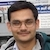# NAEST 2018 Screening Test: Double Cone Rolling UphillBy

A double cone is made by joining two right circular cones at their bases. The double cone moves up when it is placed near the base of an inclined triangular track. The upward motion of the double cone is fascinating and counter-intuitive. It appears to move against gravity. Hence it is also called anti-gravity double cone. We will explore this counter-intuitive motion of the double cone.

Question: As the double cone moves up the track,

1. The point A on the handle goes down
2. The center of mass B goes down
3. The instantaneous topmost point C goes down
4. The instantaneous bottommost point D goes down

Solution: Let us have a closer look at the triangular track. It consists of two rails (sticks) PQ and RS. The distance between P and R is less than the distance between Q and S. Thus, rails PQ and RS are not parallel to each other. The ends (P,R) are at a height $h_0$ from the ground level and the ends (Q,S) are at a greater height $h_0+h$ above the ground level. A cylindrical body released from the upper ends (Q,S) rolls down the track towards the lower ends (P,R).The double cone does not move when it is released from the upper ends (Q,S). However, when it is released from the lower ends (P,R), it starts moving towards the upper ends (R,S).

We know that objects move from an upper level to a lower level due to gravitational force. This statement is true if objects are considered as particles (point masses). For rigid bodies, the statement is true for the centre of mass of the body. Other points on the body may be moving up or down.

The double cone has an axis of symmetry that joins the vertices of two cones. It also has a plane of symmetry (the interface containing bases of two cones). The centre of mass G of the double cone lies at a point where the axis of symmetry intersects the plane of symmetry.Let $r$ be the radius of the cone's circular base. When the cone is at the lower end (P,R), its centre of mass G is at a height $h_i=h_0+r$ above the ground. The centre of mass of the cone starts coming down as it moves from the lower end to the upper end. At the upper end, centre of mass G is at a height $h_i=h_0+h$. The track and double cone are made in such a way that $r>h$. Thus, the initial height $h_i$ is greater than the final height $h_f$.The centre of mass G of the double cone moves from a higher level to a lower level because $h_i>h_f$. The motion looks counter-intuitive but it is in agreement with the laws of physics - the centre of mass of the object moves down.